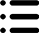#1 35% H2O2 1 份水（ 35/2= 17.5% 过氧化氢

1 35% H2O2 2 份水是 35/3 = 11.7% 过氧化氢

1 35% H2O2 3 份水是 35/4 = 8.75% 过氧化氢

1 35% H2O2 4 份水是 35/5 = 7% 过氧化氢

1 35% H2O2 5 份水是 35/6 = 5.83% 过氧化氢

1 35% H2O2 6 份水是 35/7 = 5% 过氧化氢

1 35% H2O2 7 份水是 35/8 = 4.4% 过氧化氢

1 35% H2O2 8 份水是 35/9 = 3.9% 过氧化氢

1 35% H2O2 9 份水是 35/10 = 3.5% 过氧化氢

1 35% H2O2 10 份水是 35/11 = 3.18% 过氧化氢

1 35% H2O2 13 份水是 35/14 = 2.5% 过氧化氢

1 35% H2O2 34 份水是 35/35 = 1% 过氧化氢

1 35% H2O2 69 份水是 35/70 = 0.5% 过氧化氢

1 34% H2O2 4 份水 = 6.8% 过氧化氢

1 34% H2O2 4.5 份水 = 6% 过氧化氢

1 34% H2O2 8 份水 = 3.7% 过氧化氢

1 34% H2O2 9 份水 = 3.4% 过氧化氢

1 34% H2O2 10 份水 = 3% 过氧化氢

1 29% H2O2 1 份水 = 14.5% 过氧化氢

1 29% H2O2 2 份水 = 9.6% 过氧化氢

1 29% H2O2 3 份水 = 7.25% 过氧化氢

1 29% H2O2 4 份水 = 5.8% 过氧化氢

1 29% H2O2 8 份水 = 3.2% 过氧化氢

1 29% H2O2 9 份水 = 2.9% 过氧化氢

1 27% H2O2 1.5 份水 = 10.8% 过氧化氢

1 27% H2O2 8 份水 = 3% 过氧化氢

1 10% H2O2 0.5 份水 (10/1.5) = 6.6% 过氧化氢

1 10% H2O2 2 份水 (10/3) = 3.3% 过氧化氢

1 10% H2O2 2.5 份水 (10/3.5) = 2.85% 过氧化氢

1 6% H2O2 1 份水 (6/2) = 3% 过氧化氢

1 6% H2O2 2 份水 (6/3) = 2% 过氧化氢

1 6% H2O2 3 份水 (6/4) = 1.5% 过氧化氢

1 6% H2O2 4 份水 (6/5) = 1.2% 过氧化氢

1 6% H2O2 5 份水 (6/6) = 1% 过氧化氢

1 3% H2O2 1 份水 (3/2) – 1.5% 过氧化氢

1 3% H2O2 2 份水 (3/3) = 1% 过氧化氢

1 3% H2O2 5 份水 (3/6) = 0.5% 过氧化氢

1 3% H2O2 14 份水 (3/15) = 0.2% 过氧化氢

1 3% H2O2 29 份水 (3/30) = 0.1% 过氧化氢

1 3% H2O2 69 份水 (3/70) = 0.04% 过氧化氢

苏州阿尔法阿生物 提供的实验室消毒剂主要有：3%过氧化氢消毒液6%过氧化氢消毒液，乙醇消毒液，异丙醇消毒液，复方季铵盐消毒液、复方过氧化物消毒液，工业消毒液等，更多关于过过氧化氢消毒液常用配制浓度相关问题  0512-62956104或18934597460.